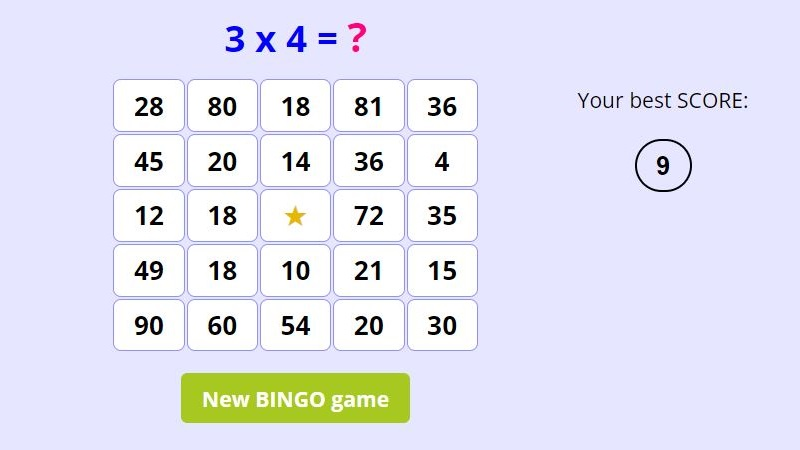Page No. 1095

# Multiplication BINGO game

Play multiplication BINGO online games. Download free printable multiplication BINGO in PDF. Get free multiplication BINGO cards. Enjoy free BINGO multiplication games. times table bingo online. online bingo multiplication games. Printable multiplication bingo game printable free. multiplication bingo printables. math multiplication bingo generator. virtual multiplication bingo free printable. multiplication bingo sheets. times table bingo printable free. printable multiplication bingo calling cards PDF. Free multiplication bingo free. multiplication bingo card generator. Learn how to play multiplication bingo game online. timestables bingo PDF. multiplication games printable bingo. math bingo multiplication.

?

B I N G O

New BINGO game

Multiply two numbers and click on the correct answer. The lower the SCORE the better.

## Multiplication Bingo Game ONLINEThere is a multiplication problem above the table. You have to click the number which represents the correct answer. The object of this multiplication BINGO game is to get five stars in a column, row or diagonally. The correct answer of the multiplication problem can be always found in the BINGO table.

## How to play multiplication BINGO online games?

Click on the correct answer of the multiplication problem written above the table. For example, we are looking for the result of the multiplication problem 3x4. The correct answer is 12, so click on the number 12 in the multiplication BINGO chart.

If your answer is correct, the number changes to a star , if your answer isn´t correct, the number will flash red and you have to click an another number.

The centre of the multiplication BINGO table is already pre-filled with a star.In this multiplication BINGO online game the result 12 corresponds to both multiplication problems: 2x6 and 3x4.

## Free multiplication BINGO printables

Download these free printable multiplication BINGO sheets. You can generate as many different multiplication BINGO printables as you want. Every child in the classroom will have own multiplication BINGO chart.

You can play our printable multiplication BINGO game with 10-sided dice or use our printable multiplication BINGO cards which you can find bellow.

This no-prep BINGO multiplication game covers multiplication facts from 1x2 through 10x10. Instuctions are included too.

Each multiplication Bingo sheet has a grid of numbers arranged in rows and columns, each cell contains an answer of multiplication problem.

How to play multiplication BINGO game:

1. First, the teacher or the parent randomly selects a multiplication flash card and reads out a multiplication problem. You can also use a 10-sided dice.
2. If the result of multiplication matches any of the numbers in the table, mark that box with a star or cross it out. See the tutorial on YouTube here.
3. If no number in the BINGO chart corresponds to the result of multiplication fact, then the player skips a turn.
4. The aim of the game is to get five marked fields in a row (horizontally, vertically, or diagonally). The central square is counted as marked.
5. Players continue to mark their BINGO sheets as the teacher reads out problems. The first player who has five stars calls out "BINGO!" and is declared the winner.

Browse all our free printable multiplication games here.

## Printable multiplication BINGO cards PDF

Download free printable multiplication BINGO cards in PDF. These free multiplication BINGO calling cards with answers on back are easy to print. Fold times table BINGO cards in half and glue.

These printable multiplication BINGO cards are FREE of charge. You can´t sell them as your own. Thanks that you share my multiplication BINGO cards printable. Please, direct people to this web page, don´t link directly to the PDF file.

## Math BINGO multiplication game

Want to deepen your kids' understanding of multiplication? Challenge them to use their knowledge of the multiplication facts to win this fun multiplication online game!

### Online multiplication BINGO games

The goal of the multiplication BINGO math game is to get the lowest possible score. It is the number of clicks you have done to gain BINGO. The best SCORE can be 4.